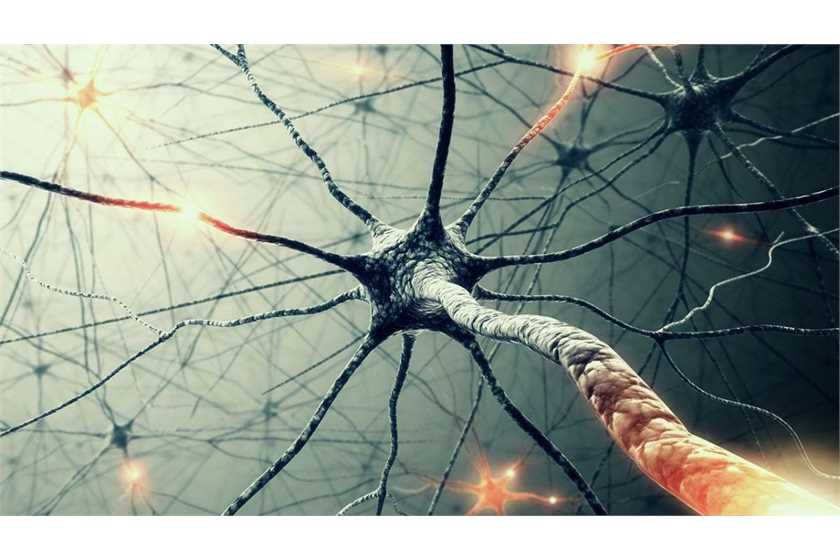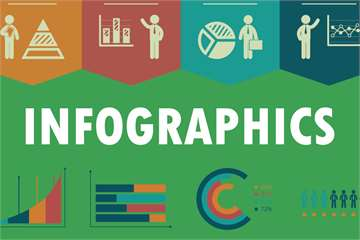# What is a genetic algorithm? How it is work?

1,264 Views Updated: 03 Nov 2016Genetic Algorithm is a method implemented in the optimization of solutions to problems by a procedure which is analogous to the biological process of evolution. Applicable for both continuous and discontinuous problems, the genetic algorithm as a methodology continuously monitors and implements changes in the pool of solutions.

For every step of selection, each solution from a previous step serves as the template for the solution in the next step, allowing the process of optimization to adopt a multistage mechanism of action. With every subsequent step in the protocol, genetic algorithm leads the path towards a perfectly optimized solution through multiple transformations in the process of achieving the end results.

The concept of genetic algorithm was invented in the field of computers and system operations, under the influence of the theories of natural selection to achieve solutions to problems of optimization or search problems with high-quality standards. The primary advantage of working with genetic algorithms is its ability to seek solutions to problems which cannot be solved by the conventional methods or algorithms. The process of application of genetic algorithms shows a marked difference from that of classical algorithms in certain ways.

Firstly, in the classical algorithm, in a given sequence of steps, yields only one point as a representative of an ideal optimized solution in every step of action. However, in genetic algorithms, a pool of points will be selected in every step, with only one being representative of the optimized solution. Secondly, in the classical algorithm, as a part of the procedure, the next point in the subsequent step is determined probabilistically. While, in a genetic algorithm, a pool of points are randomly generated through the use of number generators in each step. In fact, the ability of genetic algorithms to be applied to non-stochastic, linear or non-differentiable problems makes it a better choice over many others for optimization of problems.

The basic components of a genetic algorithm have been discussed along a defined sequence of action:

• The problem to which the solution is to be determined

• The process of encoding relevant information from the problem

• The procedure for initializing each possible solution to the problem in the pool of data points available

• The function is to be evaluated to generate multiple points as plausible solutions to the problem in each step of action

• The selection of appropriate points closest to the optimized solution as template for the subsequent steps

• The implementation of various changes and modifications in each step of the process to maximize the quality and accuracy of the estimated results leading towards optimization

In working with genetic algorithms, the advantages are many. This concept is simplified in the sense, it is easy to be understood and thus, implemented widely, expanding the range of its application. As a multistep procedure, genetic algorithm ensures the best possible solution to the problem with the greatest accuracy from a pool of points by random selection and iterative steps, which maximizes the chances of optimization. With parallel ongoing steps, the control and execution of the process are made convenient. On account of the knowledge of the chosen problem, various transformations and changes can be introduced to improve the performance and the speed of genetic algorithms. A solution at each step serves as a template for better optimization of results in the next step until a satisfactory solution is achieved.

Like every effective procedure, genetic algorithms also have their own shortcomings. The primary area of concern is the identification of the parameters and the problem on which the procedure of genetic algorithm has to be executed and applied. Besides, there have been some discrepancies regarding the quality of speed and the performance of genetic algorithms in achieving desired results. Achieving the perfectly optimized solution is considered only relative to the other solutions, therefore, there might be some differences regarding the accuracy of end results achieved. The genetic algorithms work with randomly generated points guiding towards the optimized solution, which is a problem on its own. For certain specific problems to be addressed, other algorithms may get a higher preference than genetic algorithms.

The applicability of genetic algorithms is particularly relevant in dealing with problems which yield complex results at a low pace with a certain alternative protocol. When a separate tool may be needed to have an insight into new approaches for studying problems, the genetic algorithm is the best choice. The benefits of this technology are applicable in addressing some key problems which might be similar to the ones solved previously by the application of a genetic algorithm.

#### Related polls### Compare A Online Piano Course To A Private Piano Teacher### Which is the Best Tool for Creating Infographics easily?

44 Opinions